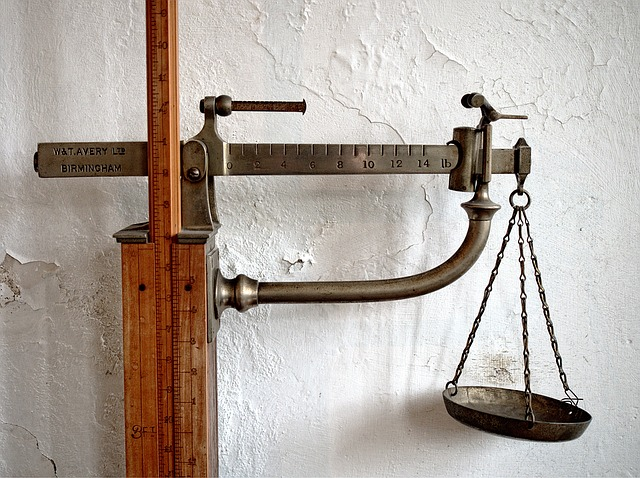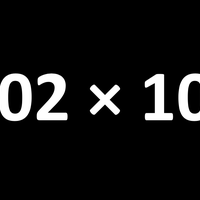# StoichiometryChemists often need to measure the quantities of elements and compounds reacting. Stoichiometry means measuring elements. Atoms of different elements (and sometimes the same element too) have different masses, so we need to take that into account when calculating reacting masses. The 'big numbers' that we put in front of formulae in chemical equations are the stoichiometric coefficients. Stoichiometry involves the concept of the mole.

#### Particulate matter and chemical change

Writing formulae and chemical equations is a fundamental skill of a chemist (it is also a universal language).

#### The mole concept

A mole is simply a number, specifically Avogadro's number (L) which is 6.02 ×1023. It is the number of atoms in exactly 12g of pure carbon-12.

#### Reacting masses and volumes

There is not a great deal of knowledge to learn in order to be confident with mole calculations, but you may well need lots of practice.

•#### Particulate matter and chemical change

Topic 1.1 Introducing some basic concepts.

•#### Reacting masses and volumes

Topic 1.3 Lots of 'moles questions'; understanding ratios is the key.

•#### The mole concept

Topic 1.2 Explaining what a mole is (spoiler: it's just a number).